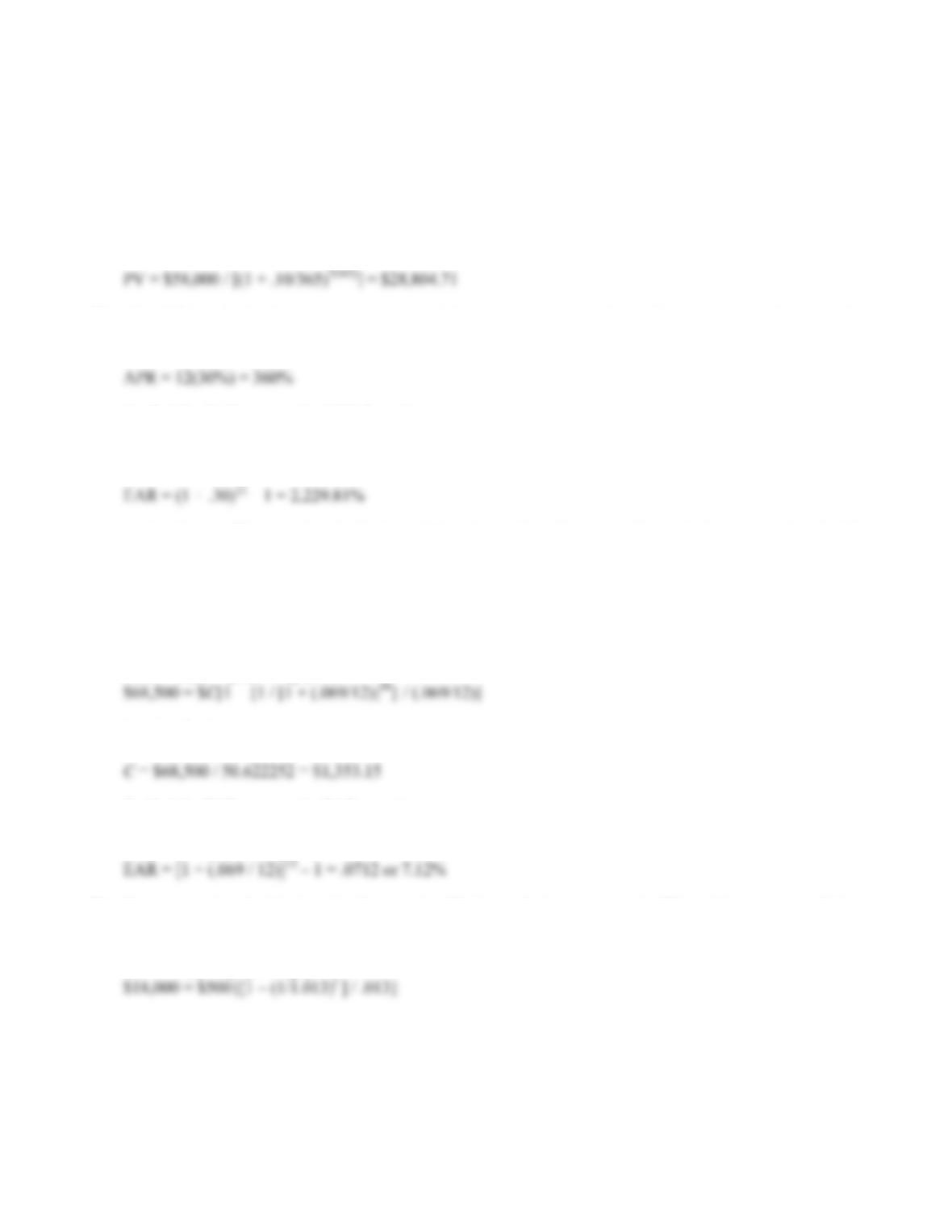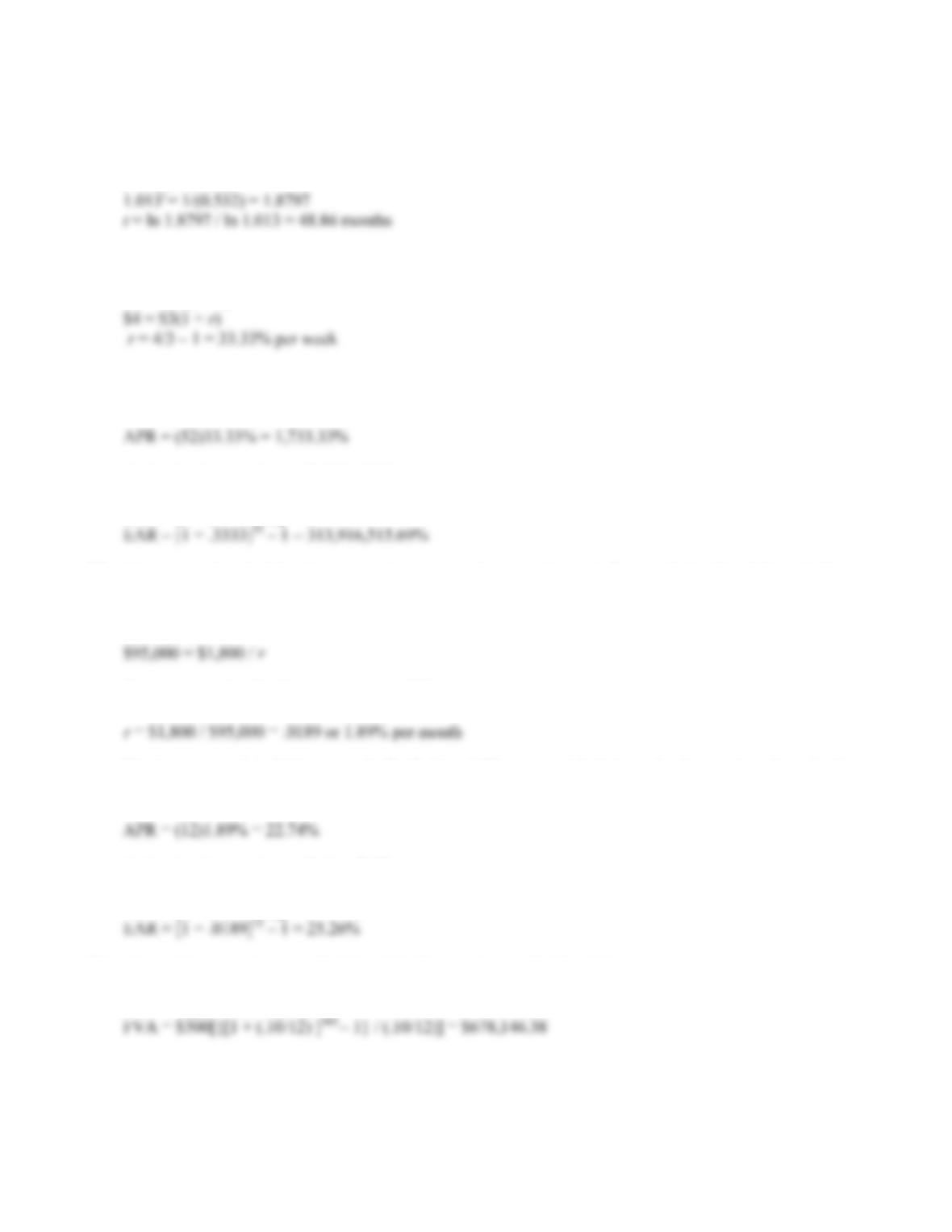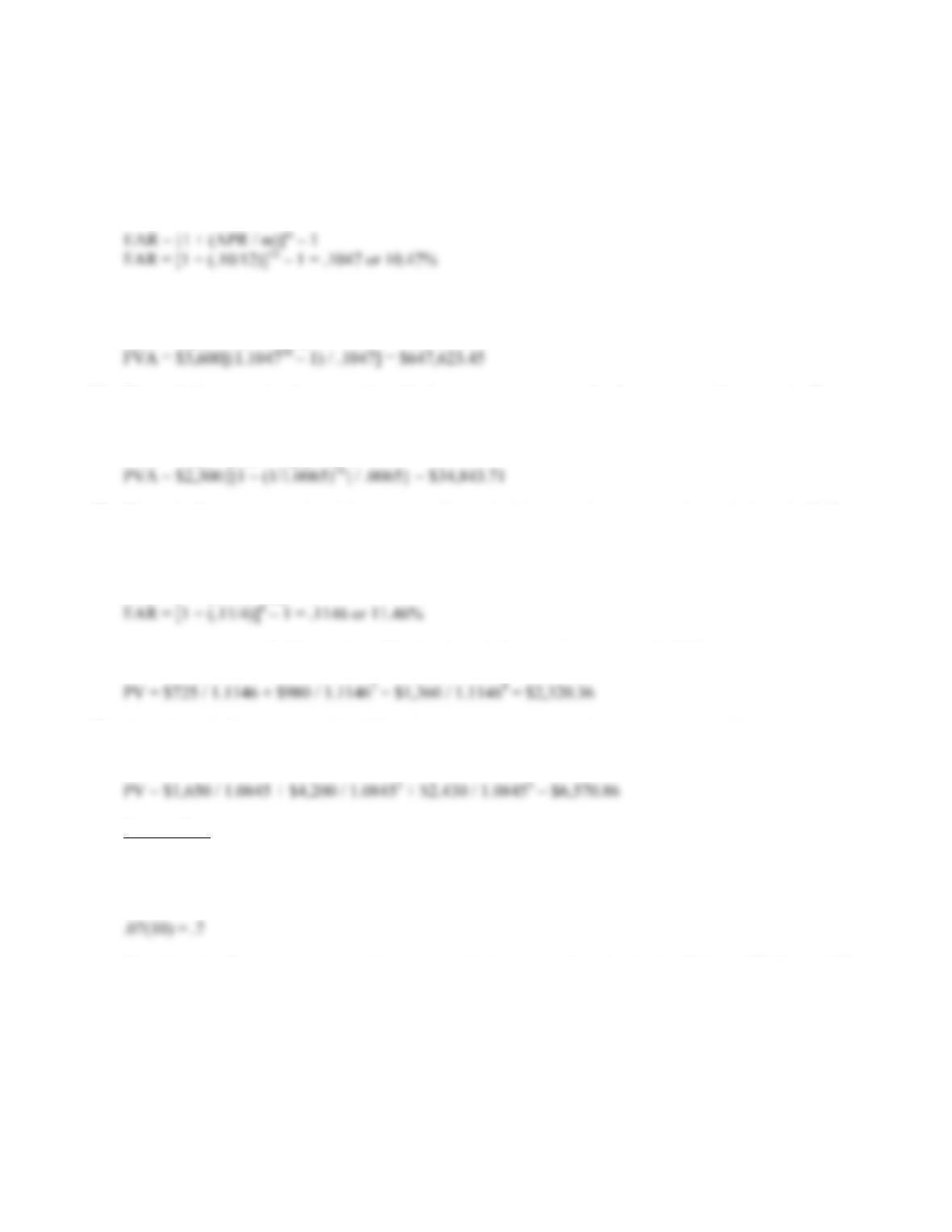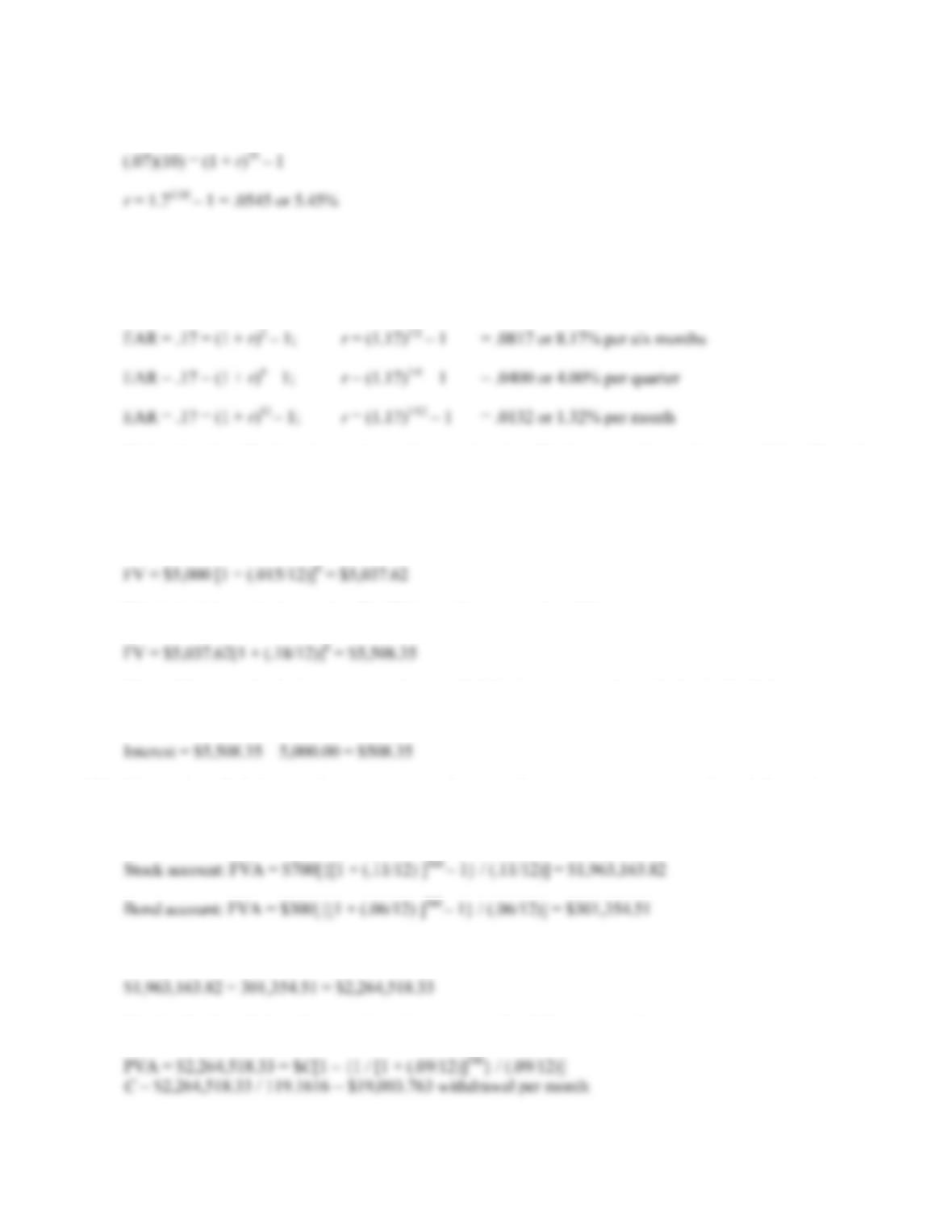# 978-0073382395 Chapter 6 Questions and Problems 21-31

Document Type
Homework Help
Book Title
Fundamentals of Corporate Finance Standard Edition 9th Edition
Authors
Stephen RossB-74 SOLUTIONS
18. For this problem, we simply need to find the PV of a lump sum using the equation:
PV = FV / (1 + r)t
It is important to note that compounding occurs daily. To account for this, we will divide the interest rate
by 365 (the number of days in a year, ignoring leap year), and multiply the number of periods by 365.
Doing so, we get:
19. The APR is simply the interest rate per period times the number of periods in a year. In this case, the
interest rate is 30 percent per month, and there are 12 months in a year, so we get:
To find the EAR, we use the EAR formula:
EAR = [1 + (APR / m)]m – 1
Notice that we didn’t need to divide the APR by the number of compounding periods per year. We do this
division to get the interest rate per period, but in this problem we are already given the interest rate per
period.
20. We first need to find the annuity payment. We have the PVA, the length of the annuity, and the interest
rate. Using the PVA equation:
PVA = C({1 – [1/(1 + r)]t } / r)
Solving for the payment, we get:
To find the EAR, we use the EAR equation:
EAR = [1 + (APR / m)]m – 1
21. Here we need to find the length of an annuity. We know the interest rate, the PV, and the payments. Using
the PVA equation:
PVA = C({1 – [1/(1 + r)]t } / r)CHAPTER 6 B-75
Now we solve for t:
1/1.013t = 1 – {[(\$18,000)/(\$500)](.013)}
1/1.013t = 0.532
22. Here we are trying to find the interest rate when we know the PV and FV. Using the FV equation:
FV = PV(1 + r)
The interest rate is 33.33% per week. To find the APR, we multiply this rate by the number of weeks in a
year, so:
And using the equation to find the EAR:
EAR = [1 + (APR / m)]m – 1
23. Here we need to find the interest rate that equates the perpetuity cash flows with the PV of the cash flows.
Using the PV of a perpetuity equation:
PV = C / r
We can now solve for the interest rate as follows:
The interest rate is 1.89% per month. To find the APR, we multiply this rate by the number of months in a
year, so:
And using the equation to find an EAR:
EAR = [1 + (APR / m)]m – 1
24. This problem requires us to find the FVA. The equation to find the FVA is:
FVA = C{[(1 + r)t – 1] / r}B-76 SOLUTIONS
25. In the previous problem, the cash flows are monthly and the compounding period is monthly. This
assumption still holds. Since the cash flows are annual, we need to use the EAR to calculate the future
value of annual cash flows. It is important to remember that you have to make sure the compounding
periods of the interest rate is the same as the timing of the cash flows. In this case, we have annual cash
flows, so we need the EAR since it is the true annual interest rate you will earn. So, finding the EAR:
Using the FVA equation, we get:
FVA = C{[(1 + r)t – 1] / r}
26. The cash flows are simply an annuity with four payments per year for four years, or 16 payments. We can
use the PVA equation:
PVA = C({1 – [1/(1 + r)]t } / r)
27. The cash flows are annual and the compounding period is quarterly, so we need to calculate the EAR to
make the interest rate comparable with the timing of the cash flows. Using the equation for the EAR, we
get:
EAR = [1 + (APR / m)]m – 1
And now we use the EAR to find the PV of each cash flow as a lump sum and add them together:
28. Here the cash flows are annual and the given interest rate is annual, so we can use the interest rate given.
We simply find the PV of each cash flow and add them together.
Intermediate
29. The total interest paid by First Simple Bank is the interest rate per period times the number of periods. In
other words, the interest by First Simple Bank paid over 10 years will be:
First Complex Bank pays compound interest, so the interest paid by this bank will be the FV factor of \$1,
or:
(1 + r)10CHAPTER 6 B-77
Setting the two equal, we get:
30. Here we need to convert an EAR into interest rates for different compounding periods. Using the equation
for the EAR, we get:
EAR = [1 + (APR / m)]m – 1
Notice that the effective six month rate is not twice the effective quarterly rate because of the effect of
compounding.
31. Here we need to find the FV of a lump sum, with a changing interest rate. We must do this problem in two
parts. After the first six months, the balance will be:
FV = \$5,000 [1 + (.015/12)]6 = \$5,037.62
This is the balance in six months. The FV in another six months will be:
FV = \$5,037.62[1 + (.18/12)]6 = \$5,508.35
The problem asks for the interest accrued, so, to find the interest, we subtract the beginning balance
from the FV. The interest accrued is:
Interest = \$5,508.35 – 5,000.00 = \$508.35
32. We need to find the annuity payment in retirement. Our retirement savings ends and the retirement
withdrawals begin, so the PV of the retirement withdrawals will be the FV of the retirement savings. So,
we find the FV of the stock account and the FV of the bond account and add the two FVs.
Stock account: FVA = \$700[{[1 + (.11/12) ]360 – 1} / (.11/12)] = \$1,963,163.82
Bond account: FVA = \$300[{[1 + (.06/12) ]360 – 1} / (.06/12)] = \$301,354.51
So, the total amount saved at retirement is:
\$1,963,163.82 + 301,354.51 = \$2,264,518.33
Solving for the withdrawal amount in retirement using the PVA equation gives us:
PVA = \$2,264,518.33 = \$C[1 – {1 / [1 + (.09/12)]300} / (.09/12)]
C = \$2,264,518.33 / 119.1616 = \$19,003.763 withdrawal per month

## Trusted by Thousands ofStudents

Here are what students say about us.